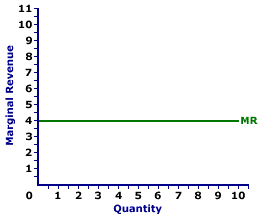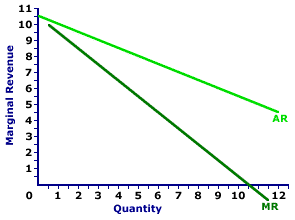Wednesday  January 26, 2022
 AmosWEB means Economics with a Touch of Whimsy!GOLD CERTIFICATE: Paper currency issued by the U. S. Treasury from the civil war until 1933 that could be exchanged for an equal value of gold. Gold certificates were used as part of a gold standard. With the exception of collectors, gold certificates have long been out of circulation, replaced first by silver certificates, then in the past few decades by Federal Reserve notes.MARGINAL REVENUE:

The change in total revenue resulting from a change in the quantity of output sold. Marginal revenue indicates how much extra revenue a firm receives for selling an extra unit of output. It is found by dividing the change in total revenue by the change in the quantity of output. Marginal revenue is the slope of the total revenue curve and is one of two revenue concepts derived from total revenue. The other is average revenue. To maximize profit, a firm equates marginal revenue and marginal cost.
Marginal revenue is the extra revenue generated when a firm sells one more unit of output. It plays a key role in the profit maximizing decision of a firm relative to marginal cost. A firm maximizes profit by equating marginal revenue, the extra revenue generated from production, with marginal cost, the extra cost of production. If these two marginals are not equal, then profit can be increased by producing more or less output.

The relation between marginal revenue and the quantity of output produced depends on market structure. For a perfectly competitive firm, marginal revenue is equal to price and average revenue, all three of which are constant. For a monopoly, monopolistically competitive, or oligopoly firm, marginal revenue is less than average revenue and price, all three of which decrease with larger quantities of output. The constant or decreasing nature of marginal revenue is a prime indication of the market control of a firm.

Marginal revenue can be represented in a table or as a curve. For a perfectly competitive firm, the marginal revenue curve is a horizontal, or perfectly elastic, line. For a monopoly, oligopoly, or monopolistically competitive firm, the marginal revenue curve is negatively sloped.

The marginal revenue received by a firm is the change in total revenue divided by the change in quantity, often expressed as this simple equation:

 marginal revenue = change in total revenuechange quantity

Perfect Competition

Perfect competition is a market structure with a large number of small firms, each selling identical goods. Perfectly competitive firms have perfect knowledge and perfect mobility into and out of the market. These conditions mean perfectly competitive firms are price takers, they have no market control and receive the going market price for all output sold.

Marginal Revenue,
Zucchini StyleThe table to the right summarizes the marginal revenue received by a hypothetical firm, Phil the zucchini grower, for selling zucchinis in a perfectly competitive zucchini market. The first column is the quantity of zucchinis sold, ranging from 0 to 10 pounds. The second column is then the price Phil receives for selling his zucchinis, which is \$4 per pound. The third column is the total revenue Phil receives for producing and selling alternative quantities of zucchinis.

Marginal revenue in the forth column is found by dividing the change in total revenue (from the third column) by the change in quantity (from the first column). For example, when Phil increases production and sales from 4 pounds of zucchinis to 5 pounds, his total revenue increases from \$16 to \$20, an increase of \$4. As such, the marginal revenue of producing the fifth pound of zucchinis is \$4 (= \$4/1). Each value in the fourth column is calculated in the same way.

The obvious point is that marginal revenue is equal to \$4 for every extra pound of zucchinis sold. This results because price is constant at \$4 for every pound of zucchinis sold. For every extra pound of zucchinis sold by Phil, he receives an extra \$4, which is the going market price. Marginal revenue is equal to price for a perfectly competitive firm.

Marginal Revenue Curve,
Zucchini StyleMarginal revenue is commonly represented by a marginal revenue curve, such as the one labeled MR and displayed in the exhibit to the right. This particular marginal revenue curve is that for zucchini sales by Phil the zucchini grower, a presumed perfectly competitive firm.

The vertical axis measures marginal revenue and the horizontal axis measures the quantity of output (pounds of zucchinis). Although quantity on this particular graph stops at 10 pounds of zucchinis, the nature of perfect competition indicates it could easily go higher.

This curve indicates that if Phil sells the first pound of zucchinis (an increase in production from 0 to 1), then his extra revenue is \$4. However, if he sells his tenth pound (an increase in production from 9 to 10), then he also receives \$4 of extra revenue. Should he sell his hundredth pound (an increase in production from 99 to 100), then he moves well beyond the graph, but his marginal revenue remains at \$4.

Because Phil is a perfectly competitive firm, his marginal revenue curve is also his demand curve and his average revenue curve. All three curves coincide for perfect competition.

Monopoly, Oligopoly, and Monopolistic Competition

While conceptually, marginal revenue is the same for market structures like monopoly, oligopoly, and monopolistic competition, because these firms are price makers rather than price takers, there are a few key differences. Market control means these market structures face negatively-sloped demand curves. As such, the price received is not fixed, but depends on the quantity of output sold, and so too does marginal revenue.

Marginal Revenue,
Medicine StyleThe table to the right summarizes the marginal revenue received by another hypothetical firm, Feet-First Pharmaceutical. This firm owns the patent to Amblathan-Plus, the only cure for the deadly, but hypothetical, foot ailment known as amblathanitis. As the only producer of Amblathan-Plus, Feet-First Pharmaceutical is a monopoly with extensive market control, facing a negatively-sloped demand curve. To sell a larger quantity of Amblathan-Plus, Feet-First Pharmaceutical must lower the price.

The first column is the quantity of Amblathan-Plus sold, ranging from 0 to 12 ounces. The second column is the price Feet-First Pharmaceutical receives for selling this medicine, which ranges from \$4.50 to \$10.50 per ounce. The third column is the total revenue Feet-First receives for producing and selling this medicine.

Marginal revenue in the forth column is found by dividing the change in total revenue (from the third column) by the change in quantity (from the first column). For example, when Feet-First Pharmaceutical increases production and sales from 4 ounces of Amblathan-Plus to 5 ounces, its total revenue increases from \$34 to \$40, an increase of \$6. As such, the marginal revenue of producing the fifth ounce of Amblathan-Plus is \$6 (= \$6/1). Each value in the fourth column is calculated in the same way.

One obvious point is that marginal revenue decreases with the quantity of medicine produced. A second point is that marginal revenue is less than the price of Amblathan-Plus for each quantity sold. The price of the fifth ounce of Amblathan-Plus is \$8, but the marginal revenue generated by the fifth ounce is only \$6. Marginal revenue is less than price. And because price is average revenue, marginal revenue is also less than average revenue.

Marginal Revenue Curve,
Medicine StyleThe marginal revenue curve for firms with market control looks a little different than that for perfect competition. The marginal revenue curve (MR) for Feet-First Pharmaceutical is displayed in the exhibit to the right. Key to this curve is that Feet-First Pharmaceutical is a monopoly provider of Amblathan-Plus and thus faces a negatively-sloped demand curve. Larger quantities of output are only possible with lower prices.

The vertical axis measures marginal revenue and the horizontal axis measures the quantity of output (ounces of medicine). Although quantity on this particular graph stops at 12 ounces of medicine, it could go higher.

This exhibit displays both the marginal revenue curve (MR) and the average revenue curve (AR), which is also the demand curve. Both are negatively sloped, but the marginal revenue curve lies below the average revenue curve, which means that marginal revenue is less than average revenue (and price) for any given quantity.

Although this marginal revenue curve, and preceding table of marginal revenue numbers, is based on the production activity of Feet-First Pharmaceutical, a well-known monopoly firm, they apply to any firm with market control. Monopolistic competition and oligopoly firms that also face negatively-sloped demand curves generate comparable marginal revenue curves.

 <= MARGINAL RETURNS MARGINAL REVENUE CURVE =>Recommended Citation:

MARGINAL REVENUE, AmosWEB Encyclonomic WEB*pedia, http://www.AmosWEB.com, AmosWEB LLC, 2000-2022. [Accessed: January 26, 2022].

Check Out These Related Terms...

Or For A Little Background...

And For Further Study...

Related Websites (Will Open in New Window)...
Search Again?PINK FADFLY[What's This?] Today, you are likely to spend a great deal of time wandering around the shopping mall hoping to buy either a New York Yankees baseball cap or a solid oak entertainment center. Be on the lookout for attractive cable television service repair people.Your Complete ScopeThe word "fiscal" is derived from a Latin word meaning "moneybag.""It has been my philosophy of life that difficulties vanish when faced boldly. "-- Isaac AsimovMRMarginal RevenueA PEDestrian's Guide Xtra CreditTell us what you think about AmosWEB. Like what you see? Have suggestions for improvements? Let us know. Click the User Feedback link.| | | | | | | | | | |
| | | |

Thanks for visiting AmosWEB